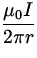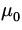## A long straight wire

We first consider a very long straight wire carrying a current I . In Fig. 1.6 we illustrate this for a wire with current coming out of the page.At a distance r from the wire, as shown, the magnitude of the magnetic field is given by

 B =, (6)

where the constantis called the permeability of free space, and is given (exactly) by= 4x 10- 7 Tm / A . (7)

We will see shortly the reason why this is an exact number. The direction of the magnetic field of this wire is indicated in the above figure, and can be remembered by the following rule:
Point the thumb of your right hand in the direction of the current along the wire - the curl of your fingers then indicates the direction of the magnetic field.Next: A circular loop Up: Sources of the Magnetic Previous: Sources of the Magnetic